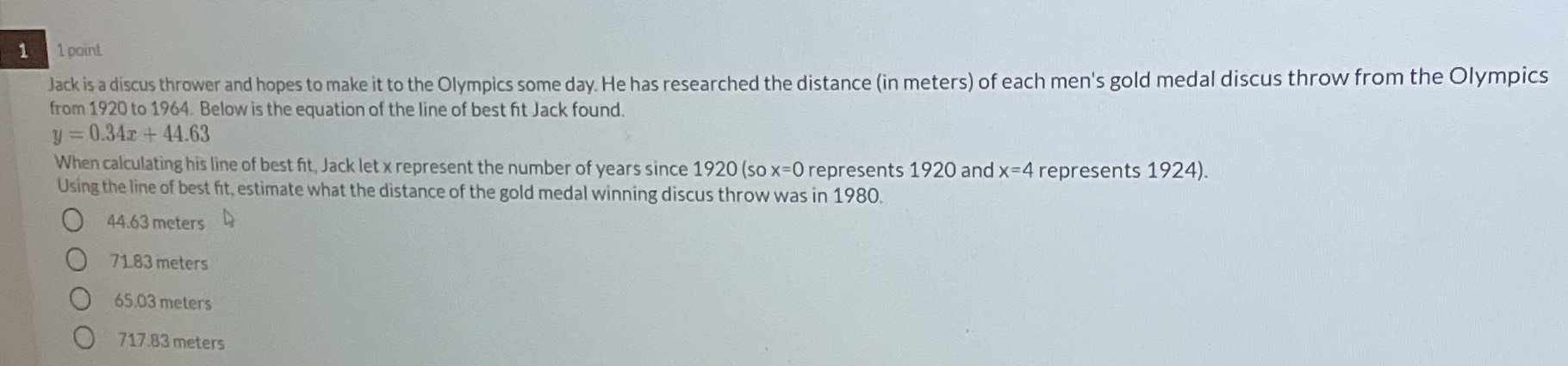### ¿Todavía tienes preguntas de matemáticas?

Pregunte a nuestros tutores expertos
Algebra
Pregunta$$1$$ 1 point. Jack is a discus thrower and hopes to make it to the Olympics some day. He has researched the distance (in meters) of each men's gold medal discus throw from the Olympics from $$1920$$ to $$1964$$ . Below is the equation of the line of best fit Jack found.

$$y = 0.34 x + 44.63$$

When calculating his line of best fit, Jack let x represent the number of years since $$1920$$ (so $$x = 0$$ represents $$1920$$ and $$x = 4$$ represents $$1924$$ ). Using the line of best fit, estimate what the distance of the gold medal winning discus throw was in $$1980$$ .

$$44.63$$ meters

$$71.83$$ meters

$$65.03$$ meters

$$717.83$$ meters